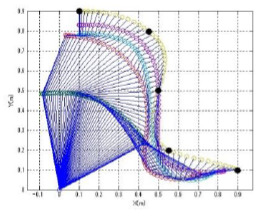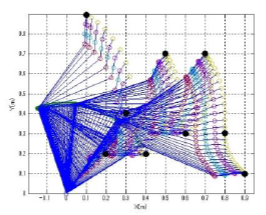# Research on Optimal Number of Joints and Lengths of Multi-Jointed Robot Arm

Author:
Hyeongjun Kim and Hiroshi Ymakawa (Waseda University)

The control and trajectory optimization problems of multi-jointed robot arms have been studied in the past, but there are only a few researches on the optimal number of joints and the optimal lengths of such robots. This study deals with the optimization (performed with modeFRONTIER) of the latter aspects, consisting in identifying the optimal number of joints and lengths of a multi-jointed robot arm and minimizing the objective functions under constraints.The robot arm is a rigid body moving horizontal plane, disregarding potential energy and dissipative energy by damping. The optimization was performed using a genetic algorithm with 100 generations. With driving energy minimization as the only objective, in case of a straight line trajectory, the optimal number of joint was 4.In case of a smooth curve trajectory and a curve trajectory with many minima the optimal number of joints was 3. The optimization has also been performed with two objectives, i.e. minimization of driving energy and maximization of the manipulability measure, the second being an index quantifying robot operation capability from the kinematic viewpoint. As a result, it was possible to select a solution with higher manipulability from the Pareto set in case of straight and smooth curve trajectories; however, in the case of a curve trajectory with many minima, it was difficult to select one solution due to the trade off relationship between the two objectives. Thus it would be necessary to add another objective function in order to determine optimal solution for complicated trajectories.